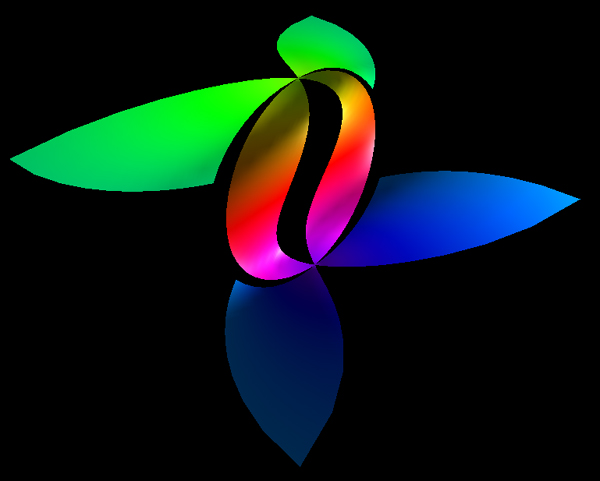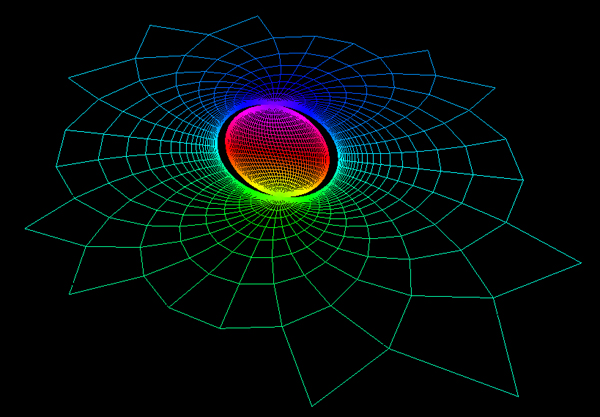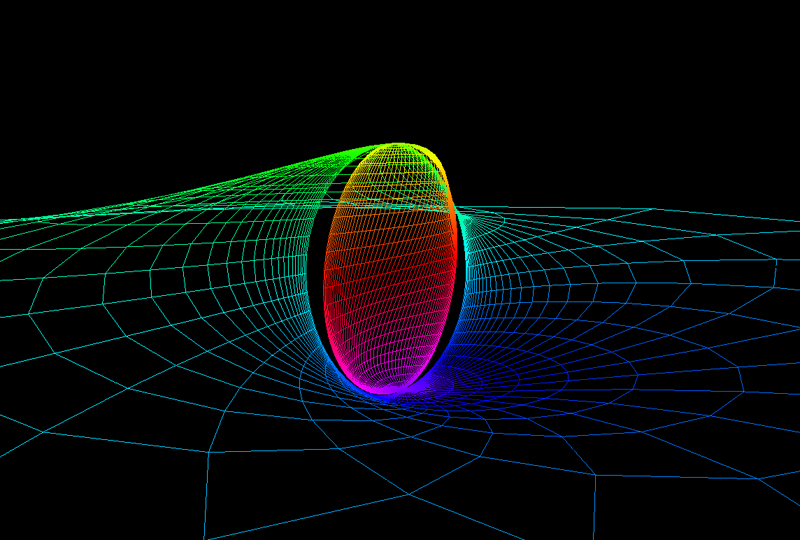# Ghost Plane

Contributed by Roger Bagula
Graphics by Paul Bourke
May 2003
x = cos(u) sinh(t) / (cosh(t) - cos(u))
y = cos(u) sin(u) / (cosh(t) - cos(u))
z = sin(u)
-pi <= u <= pi
-pi <= t <= pi